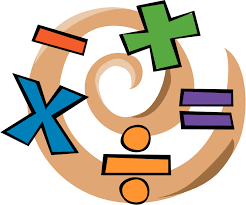Curriculum OverviewChapter 1 Numerical Expressions and Factors
Chapter 2 Fractions and Decimals
Chapter 3 Ratios and Rates
Chapter 4 Percents
Chapter 5 Algebraic Expressions and Properties
Chapter 6 Equations
Chapter 7 Area, Surface Area, and Volume
Chapter 8 Integers, Number Lines, and the Coordinate Plane
Chapter 9 Statistical Measures
Chapter 10 Data Displays